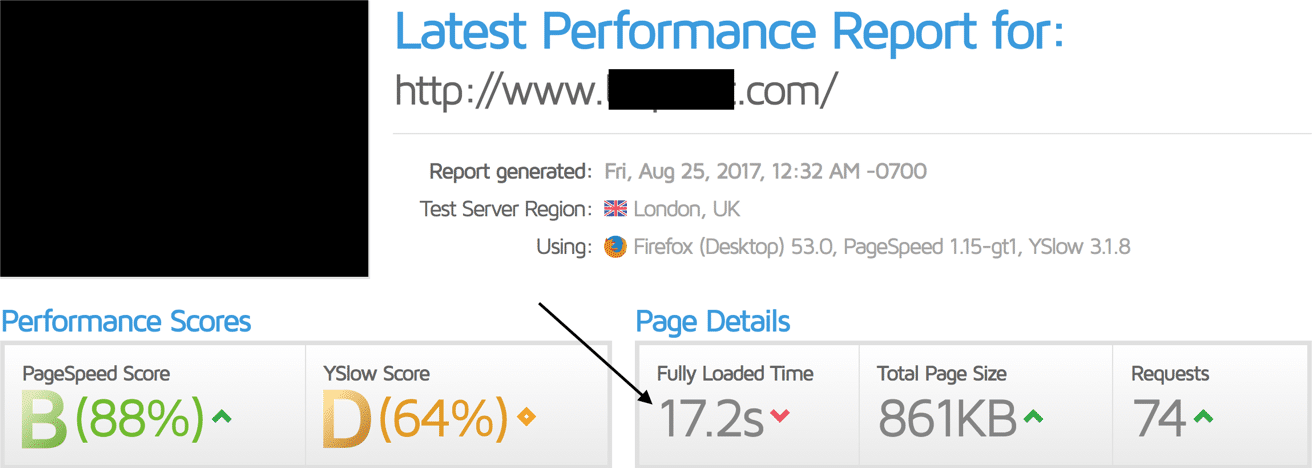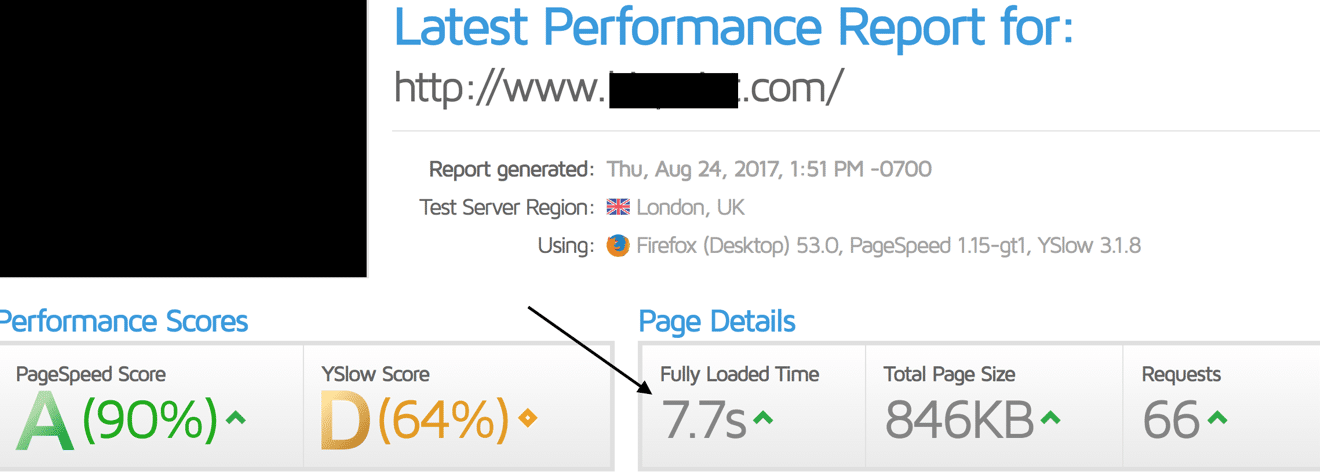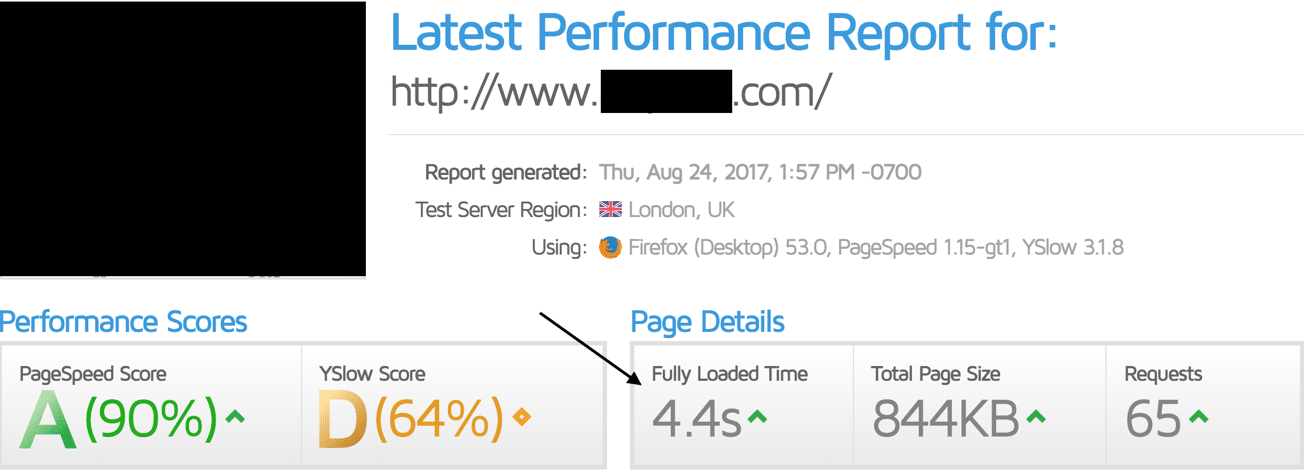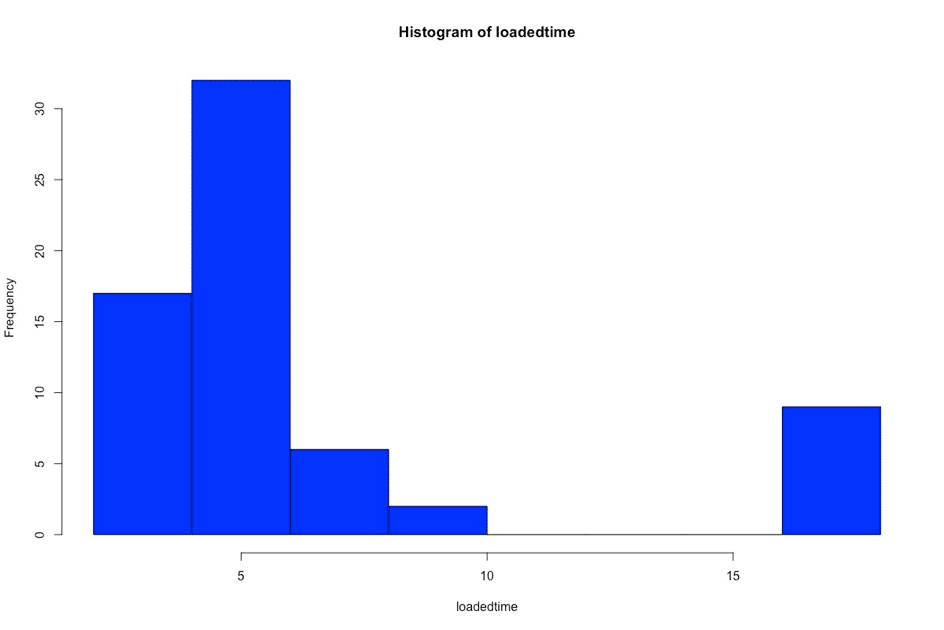Utku Demirhan
in Measurement

27 Jan 2020

## Nature of the Measurements

As a course of its nature, measurements have to be repeated both on a regular and random basis. With regularness we can be sure on; we are recording the very nature of the system that we observe and with randomness; we can be sure on we do not fool by the periodicity. Think about a barber shop if you always visit it on weekends than you might think barber shops are always busy places but most of the time in the weekdays they’re not. Now, let's go back to the site speed testing tools.

## GTMetrix Results

When we make a couple of tests on the same website from the same location with GTmetrix we can easily see calculations differs significantly from each other. Here I want to show you a couple of results:So our measurements are: 7.7, 4.4 and 17.2 From these calculations a couple of questions arise:

• Which one is my true fully loaded time in GTMetrix scale?
• If I take the mean value of these three calculations will I have the exact fully loaded time? (nearly 10)
• If I take another tree calculations will it differ from the previous ones? If so how much?
• Is 17.2 value one-time thing or will it repeat?

We can expand these questions and try to guess our real loaded time or we can repeat our measurements and use the confidence interval to obtain the most trustable mean value.

## Mean and Confidence Interval

When we repeat our measurements we start to understand the nature of the system better. We start to see if the values are discrete or continuous, we can see if the calculations differ through time or not. And most importantly we can lower our confidence interval towards the mean and get closer to our true mean value.
In this example, measurements are made from Vancouver, Canada which is default location. Let me show you how I calculate the true fully loaded time mean value for this website: First I measure the fully loaded time for this website 16 times randomly during the day.

` >loadedtime <- c(5.4,8.1,3.9,4.5,6.2,4.3,4.8,17.4,8.5,4.1,4.7,5.4,5.0,5.1,8.8,5.5)`

Then I calculated the mean, standard deviation and confidence interval values for these measurements. You can use excel kind software for all of these calculations. In excel Mean and STDEV functions will help you to calculate. You can also use this, pre-prepared excel file. Meanvalue = 6.35625 Confidance interval = 1.960 * STDEV(loadedtime) / sqrt(n); = 1.62777 So this measurements means our mean value is between 6.35625 ± 1.62777; 4.72848 and 7.98402 Not as accurate as we desired. So keep measuring. When we reach up to the 100th measurement we can check the mean and confidence interval again. 100th Measurement Meanvalue = 7.52 Confidance interval = 1.06173 Here we have a lower interval 7.52 ± 1.06173 Our true mean is between 6.45 and 8.59, we can keep measuring and lower the interval but for now, that is enough, you get the point. Here we can also see the interval is shifted. The probability of 4.72 mean value we calculated from 16 sample is not the case anymore. From the measurement I made I can easily say that values over 15 are repeating themselves. You can see it in this graph:Note: Fully load time distribution: We measure fully loaded time mostly below 10. But we also record some values above 15 but any in the middle which may state some kind of problem that we don’t know the source. You can see this is a much much better picture for your fully loaded time. So why did the mean value increased? Because when we observed 16 values we haven't been able to observe the behavior of this web site completely. After 1 or 2 days of measurements, we get the real picture here. Vancouver or London?

The next question popped-up in my mind is; server location of this website is probably closer to the London. So the mean value, will it differ from location to location? The answer lies in the mean and the confidence interval again. When I calculate the mean and interval from 16 measurements from London for this website: Mean value = 6.18125 Confidance interval = 1.960 * STDEV(loadedtime) / sqrt(n); = 2.127653  So this means our mean value is between 6.18125 ± 2.127653; 4.053597 and 8.308903 100th Measurement Meanvalue = 6.869 Confidance interval = 0.9071042 Here we have a lower interval 6.869 ± 0.9071042  It looks like London calculations have lower results than Vancouver but this sample rate is not enough to exactly say London has lower mean value because both of their mean values sit inside each other. Increasing the sample rate we can solve this issue and most probably we can see that London has lower fully loaded time.

## Final

When you want to know the fully loaded time of your website for sure you need to use mean value and confidence interval. Confidence interval tells you how accurate your mean value is. You can also consider using GTmetrix or Pingdom Pro to record your URLs on regular basis to understand their true loaded time. I know even basic statistics looks complicated but if you want to measure your loaded time correctly then you need to follow this process. Feel free to ask me anything on this.